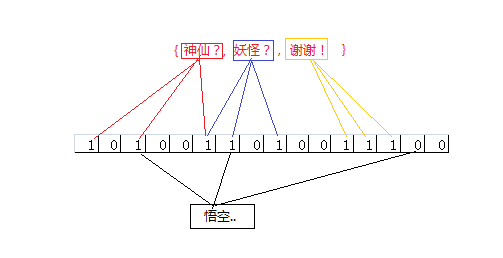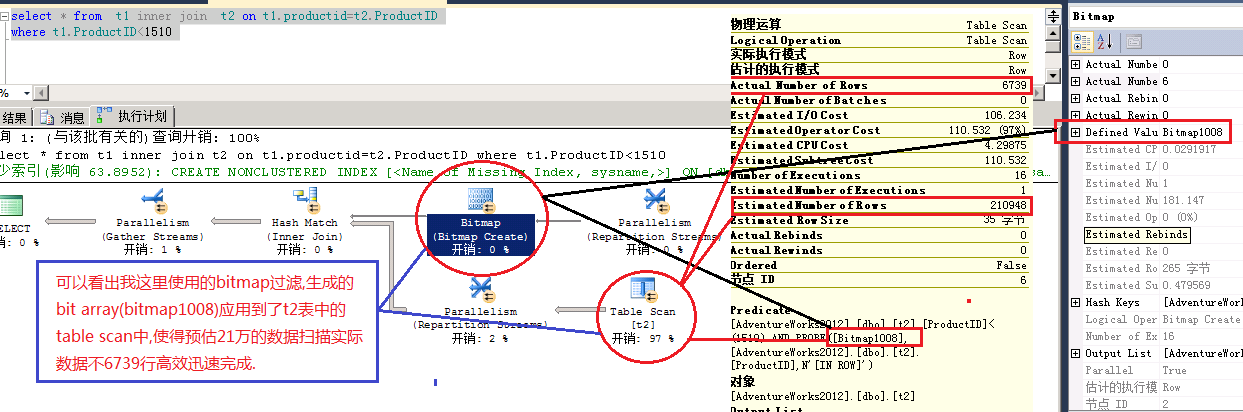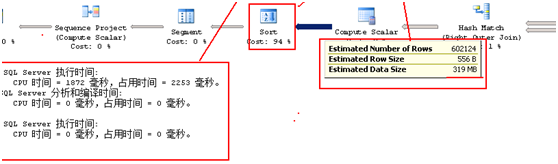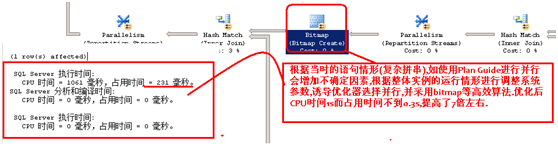# SQL Server优化器特性-位图过滤(Bitmap)

http://en.wikipedia.org/wiki/Bitmap_index

SQL Server的位图过滤采用的布隆过滤(bloom filter)方式,这里我简单说下布隆过滤的实现方式.图1-1```USE AdventureWorks
GO

SELECT
p.ProductID + (a.number * 1000) AS ProductID,
p.Name + CONVERT(VARCHAR, (a.number * 1000)) AS Name,
p.ProductNumber + ‘-‘ + CONVERT(VARCHAR, (a.number * 1000)) AS ProductNumber,
p.MakeFlag,
p.FinishedGoodsFlag,
p.Color,
p.SafetyStockLevel,
p.ReorderPoint,
p.StandardCost,
p.ListPrice,
p.Size,
p.SizeUnitMeasureCode,
p.WeightUnitMeasureCode,
p.Weight,
p.DaysToManufacture,
p.ProductLine,
p.Class,
p.Style,
p.ProductSubcategoryID,
p.ProductModelID,
p.SellStartDate,
p.SellEndDate,
p.DiscontinuedDate
INTO T1
FROM Production.Product AS p
CROSS JOIN master..spt_values AS a
WHERE
a.type = ‘p‘
AND a.number BETWEEN 1 AND 50
GO

SELECT
ROW_NUMBER() OVER
(
ORDER BY
x.TransactionDate,
(SELECT NEWID())
) AS TransactionID,
p1.ProductID,
x.TransactionDate,
x.Quantity,
CONVERT(MONEY, p1.ListPrice * x.Quantity * RAND(CHECKSUM(NEWID())) * 2) AS ActualCost
INTO T2
FROM
(
SELECT
p.ProductID,
p.ListPrice,
CASE
WHEN p.productid % 26 = 0 THEN 26
WHEN p.productid % 25 = 0 THEN 25
WHEN p.productid % 24 = 0 THEN 24
WHEN p.productid % 23 = 0 THEN 23
WHEN p.productid % 22 = 0 THEN 22
WHEN p.productid % 21 = 0 THEN 21
WHEN p.productid % 20 = 0 THEN 20
WHEN p.productid % 19 = 0 THEN 19
WHEN p.productid % 18 = 0 THEN 18
WHEN p.productid % 17 = 0 THEN 17
WHEN p.productid % 16 = 0 THEN 16
WHEN p.productid % 15 = 0 THEN 15
WHEN p.productid % 14 = 0 THEN 14
WHEN p.productid % 13 = 0 THEN 13
WHEN p.productid % 12 = 0 THEN 12
WHEN p.productid % 11 = 0 THEN 11
WHEN p.productid % 10 = 0 THEN 10
WHEN p.productid % 9 = 0 THEN 9
WHEN p.productid % 8 = 0 THEN 8
WHEN p.productid % 7 = 0 THEN 7
WHEN p.productid % 6 = 0 THEN 6
WHEN p.productid % 5 = 0 THEN 5
WHEN p.productid % 4 = 0 THEN 4
WHEN p.productid % 3 = 0 THEN 3
WHEN p.productid % 2 = 0 THEN 2
ELSE 1
END AS ProductGroup
FROM bigproduct p
) AS p1
CROSS APPLY
(
SELECT
transactionDate,
CONVERT(INT, (RAND(CHECKSUM(NEWID())) * 100) + 1) AS Quantity
FROM
(
SELECT
NTILE(p1.ProductGroup) OVER
(
ORDER BY number
) AS groupRange
FROM master..spt_values
WHERE
type = ‘p‘
) AS z
WHERE
z.groupRange % 2 = 1
) AS x```
View Code

```select * from  t1 inner join  t2 on t1.productid=t2.ProductID
where t1.ProductID<1510```图1-2

http://www.oracle.com/technetwork/articles/sharma-indexes-093638.html

关于布隆过滤器的使用可以参考wikipedia

http://en.wikipedia.org/wiki/Bloom_filter图2-1图2-2

Plan Guide, Trace Flag , cost threshold for parallelism

/*******************************************************************/